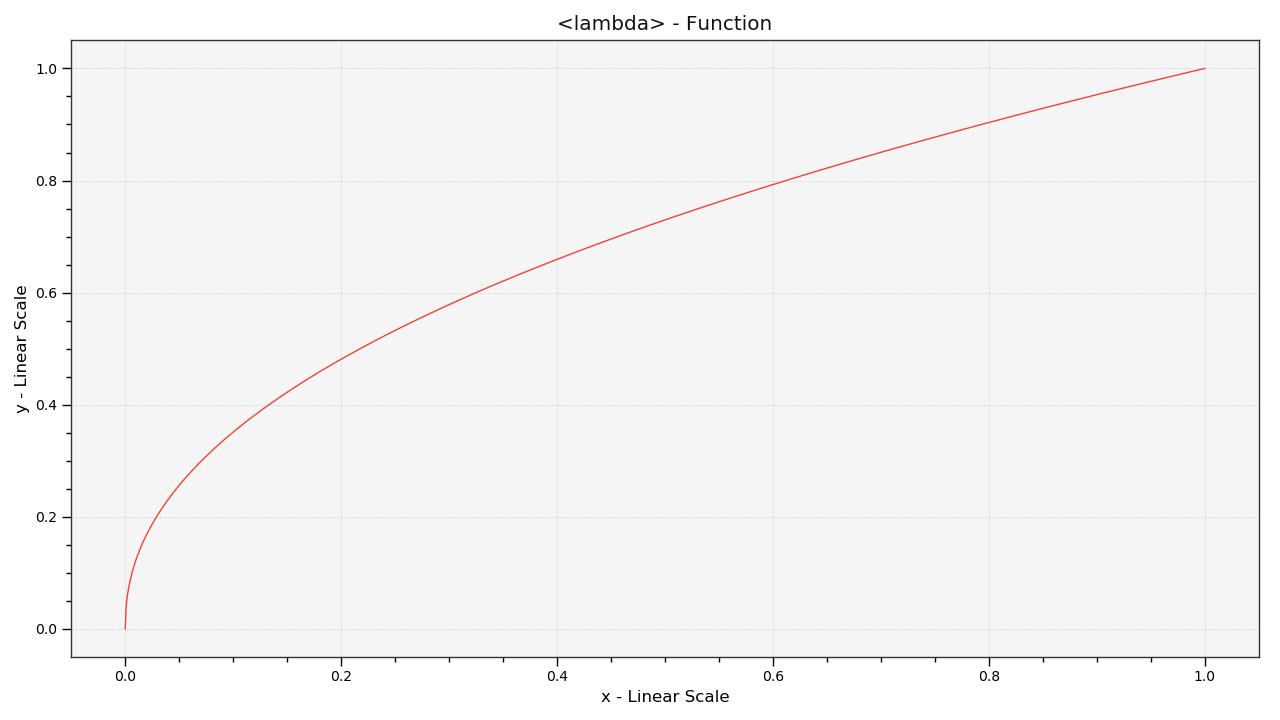# colour.plotting.plot_single_function¶

colour.plotting.plot_single_function(function, samples=None, log_x=None, log_y=None, **kwargs)[source]

Plots given function.

Parameters: Other Parameters: function (callable, optional) – Function to plot. samples (array_like, optional,) – Samples to evaluate the functions with. log_x (int, optional) – Log base to use for the x axis scale, if None, the x axis scale will be linear. log_y (int, optional) – Log base to use for the y axis scale, if None, the y axis scale will be linear. **kwargs (dict, optional) – {colour.plotting.artist(), colour.plotting.plot_multi_functions(), colour.plotting.render()}, Please refer to the documentation of the previously listed definitions. Current figure and axes. tuple

Examples

>>> plot_single_function(partial(gamma_function, exponent=1 / 2.2))
... # doctest: +SKIP